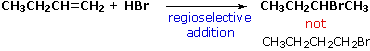# How can I apply Markovnikov’s rule in CH_3CH_2CH=CH2 + HBr -> to determine which carbon the hydrogen bonds with and which carbon the bromine atom bonds with?

Jun 25, 2015

Add the $\text{H}$ atom to the alkene carbon that has more $\text{H}$ atoms.

#### Explanation:

The official statement of Markovnikov's rule is, "When an unsymmetrical reagent adds to an unsymmetrical alkene, the more positive part of the reagent adds to the less substituted carbon atom of the alkene".

In plain language this means that if the reagent is $\text{H-X}$, the $\text{H}$ adds to the carbon with more $\text{H}$ atoms.

So the $\text{H}$ adds to $\text{C-1}$ and the $\text{Br}$ adds to $\text{C-2}$.The reason is that the ${\text{H}}^{+}$ from the $\text{HBr}$ adds to the carbon that will give the more stable carbocation.

${\text{CH"_3"CH"_2"CH=CH"_2 stackrel("H"^+)(→) underbrace("CH"_3"CH"_2stackrel(+)("C")"H-CH"_2"-H")_color(red)("2° carbocation") stackrel("Br"^(-))(→) "CH"_3"CH"_2"CHBr-CH}}_{3}$

If the ${\text{H}}^{+}$ adds to $\text{C-1}$, the intermediate is the more stable 2° carbocation.

If the ${\text{H}}^{+}$ adds to $\text{C-2}$, the intermediate is the less stable 1° carbocation.

$\text{CH"_3"CH"_2"CH=CH"_2 stackrel("H"^+)(→) underbrace("CH"_3"CH"_2"CH"_2"-"stackrel(+)("C")"H"_2)_color(red)("1° carbocation") stackrel("Br"^(-))(→) "CH"_3"CH"_2"CH"_2"-CH"_2"-Br}$